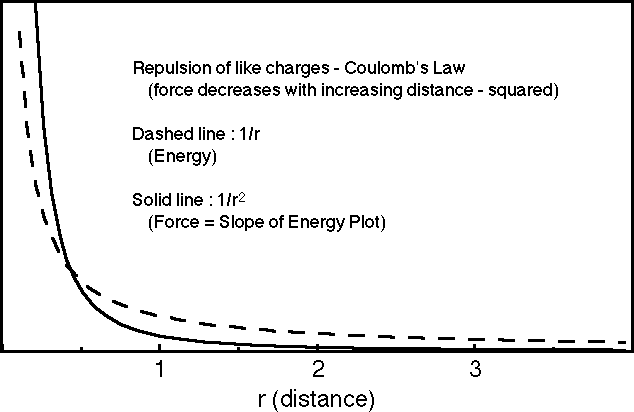What are Force Laws?

Lines-of-Force diagrams work for "inverse square Force Laws", but not all force laws are of that type.

What are Force Laws?

Force laws are formulae for reckoning the force between two objects. Familiar examples are:

 Law r (Distance) Dependance Examples [constant force] F = const force on a plow or from steady wind Hooke's Law F = const * r ideal spring approx. chemical bond Coulomb's Law F = const / r2 "inverse square" electrical charges magnetic poles gravity

Note that the most fundamental forces are "inverse square".

Think carefully about graphical representations of these forces and the corresponding energies,

remembering that F = dE / dr: